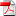# Neural networks-based backward scheme for fully nonlinear PDEs - H. Pham, X. Warin

27
Juil

We propose a numerical method for solving high dimensional fully nonlinear partial differential equations (PDEs). Our algorithm estimates simultaneously by backward time induction the solution and its gradient by multi-layer neural networks, through a sequence of learning problems obtained from the minimization of suitable quadratic loss functions and training simulations. This methodology extends to the fully non- linear case the approach recently proposed in (Huré, Pham, Warin, 2019) for semi-linear PDEs. Numerical tests illustrate the performance and accuracy of our method on several examples in high dimension with nonlinearity on the Hessian term including a linear quadratic control problem with control on the diffusion coefficient.

•RR-FIME-19-07